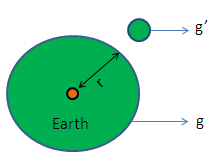Calculate Acceleration Due to Gravity on Outside Earth

# Acceleration Due to Gravity Calculation

The calculator calculates the occurrence of the acceleration due to gravity on exterior portion of the earth.

#### Formula:

g' = ( re2 / r2) * g
Where,
g - Earth Surface, re - Earth Radius, r - Outside Radius of Earth, g' - Acceleration due to gravity
 Earth's surface (g) : Earth's radius (re): Radius of outside the earth (r): Acceleration due to gravity (g'):